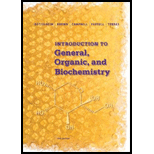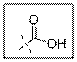# 18-4 Answer true or false. (a) The functional groups of a carboxylic acid are a carbonyl group bonded to a hydroxyl group. (b) The VSEPR model predicts bond angles of 180° about the carbonyl carbon of a carboxyl group. (c) The VSEPR model predicts bond angles of 109.5° about the oxygen of the OH group of a carboxyl group. (d) The carbonyl carbon of a carboxyl group can be a stereocenter, depending on its location within a molecule. (e) Carboxylic acids can be prepared by chromic acid oxidation of primary alcohols and of aldehydes. (f) The product of chromic acid oxidation of hexanoic acid is 1-hexanol.### Introduction to General, Organic a...

11th Edition
Frederick A. Bettelheim + 4 others
Publisher: Cengage Learning
ISBN: 9781285869759### Introduction to General, Organic a...

11th Edition
Frederick A. Bettelheim + 4 others
Publisher: Cengage Learning
ISBN: 9781285869759

#### Solutions

Chapter 18, Problem 18.4P
Textbook Problem

## 18-4 Answer true or false.(a) The functional groups of a carboxylic acid are a carbonyl group bonded to a hydroxyl group.(b) The VSEPR model predicts bond angles of 180° about the carbonyl carbon of a carboxyl group.(c) The VSEPR model predicts bond angles of 109.5° about the oxygen of the OH group of a carboxyl group.(d) The carbonyl carbon of a carboxyl group can be a stereocenter, depending on its location within a molecule.(e) Carboxylic acids can be prepared by chromic acid oxidation of primary alcohols and of aldehydes.(f) The product of chromic acid oxidation of hexanoic acid is 1-hexanol.

Expert Solution
Interpretation Introduction

(a)

Interpretation:

Whether the statement.

The functional groups of carboxylic acids are a carbonyl group bonded to a hydroxyl group is true or false.

Concept Introduction:

Carboxylic acid group contain one carbonyl group and one hydroxyl group that is −COOH.

### Explanation of SolutionAs per the given structure the carboxyl group contains a carbonyl group which is bonded to a hydroxyl group. So the statement is true.

Carboxylic acid functional group is known as COOH. When this group is elaborately written it shows two functional groups. Those groups are carbonyl group and another is hydroxyl group.

So the carboxyl group contains a carbonyl group which is bonded to a hydroxyl group.

Expert Solution
Interpretation Introduction

(b)

Interpretation:

Whether the statement.

The VSEPR model predicts bond angles of 180°C about the carbonyl carbon of a carboxyl group is true or false.

Concept Introduction:

Carboxylic acid group contain one carbonyl group and one hydroxyl group. VSEPR theory stands for valence shell electron pair repulsion theory. This theory predict the geometry of molecules on the basis of the number of electron pairs present surrounding the central atoms.

### Explanation of SolutionAs per the given structure the carboxyl group contains a carbonyl group which is bonded to a hydroxyl group. The angle is 1200. So the statement is false.

Carboxylic acid functional group is known as COOH. When this group is elaborately written it shows two functional groups. Those groups are carbonyl group and another is hydroxyl group. The hybridization of the carbonyl carbon is sp2 and thus expected bond angle is 1200.

Expert Solution
Interpretation Introduction

(c)

Interpretation:

Whether the statement.

The VSEPR model predicts bond angles of 109.50 about the oxygen of the OH group of a carboxyl group is true or false must be explained.

Concept Introduction:

Carboxylic acid group contain one carbonyl group and one hydroxyl group and the oxygen atom of hydroxyl group is sp3 hybridized. VSEPR theory stands for valence shell electron pair repulsion theory. This theory predict the geometry of molecules on the basis of the number of electron pairs present surrounding the central atoms.

### Explanation of SolutionAs per the given structure the carboxyl group contains a carbonyl group which is bonded to a hydroxyl group. The oxygen atom of hydroxyl group is sp3 hybridized. So expected bond angle is angle is 109.50

But due to two lone pair electrons on oxygen atom bond angle will be lesser than 109.50.So the statement is false.

Carboxylic acid functional group is known as COOH. When this group is elaborately written it shows two functional groups. Those groups are carbonyl group and another is hydroxyl group. The hybridization of the oxygen atom of hydroxyl group is sp3 and thus expected bond angle is 109.50. Due to lone pairs of electrons on oxygen atom the bond angle will be lesser.

Expert Solution
Interpretation Introduction

(d)

Interpretation:

Whether the statement.

The carbonyl carbon of a carboxyl group can be a stereocenter, depending on its location within a molecule is true or false.

Concept Introduction:

Carbonyl carbon has double bonded oxygen atom and also linked with hydroxyl group. Stereocentre is defined as the centre in which all the groups attached to the atom generally carbon atom, should be different.

### Explanation of Solution

As carbonyl group has double bonded oxygen atom and thus it can’t be a stereocenter. So the statement is false.

Carboxyl group is a carbonyl group bonded to a hydroxyl group. Carbonyl carbon being double bonded to oxygen it is not a stereocenter.

Expert Solution
Interpretation Introduction

(e)

Interpretation:

Whether the statement.

Carboxylic acid can be prepared by chromic acid oxidation of primary alcohols and of aldehydes is true or false.

Concept Introduction:

Chromic acid is very strong oxidizing agent. Oxidizing agent is defined as the oxidant which gains electrons and reduced in the chemical reaction.

### Explanation of Solution

Chromic acid being very strong oxidizing agent it is possible to oxidize primary alcohols and aldehydes to carboxylic acid. So the statement is true.

When primary alcohols are oxidized with chromic acid then the alcohol is first converted to aldehyde which in turn converted to carboxylic acid.

Expert Solution
Interpretation Introduction

(f)

Interpretation:

Whether the statement.

The product of chromic acid oxidation of hexanoic acid is 1-hexanol is true or false.

Concept Introduction:

When 1-hexanol is oxidized it is converted to hexanoic acid. Oxidation is the process in which either loss of electrons or increase in the oxidation number takes plcae.

### Explanation of Solution

1-Hexanol can be converted to hexanoic acid after treatment with chromic acid but the product of chromic acid oxidation of hexanoic acid is not 1-hexanol.

So the statement is false.

1-Hexanol can be converted to hexanoic acid with chromic acid. But 1-hexanol can’t be obtained after oxidizing hexanoic acid with chromic acid. For getting 1-hexanol from hexanoic acid reducing agent is required instead of chromic acid.

### Want to see more full solutions like this?

Subscribe now to access step-by-step solutions to millions of textbook problems written by subject matter experts!

### Want to see more full solutions like this?

Subscribe now to access step-by-step solutions to millions of textbook problems written by subject matter experts!